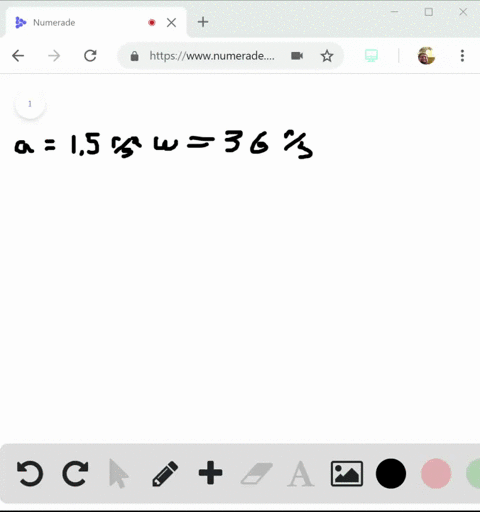Enroll in one of our FREE online STEM summer camps. Space is limited so join now!View Summer Courses### The rotating blade of a blender turns with consta…

08:12
AH
Carnegie Mellon University

Need more help? Fill out this quick form to get professional live tutoring.

Get live tutoring
Problem 10

An electric fan is turned off, and its angular velocity decreases uniformly from 500 rev/min to 200 rev/min in 4.00 s. (a) Find the angular acceleration in rev/s$^2$ and the number of revolutions made by the motor in the 4.00-s interval. (b) How many more seconds are required for the fan to come to rest if the angular acceleration remains constant at the value calculated in part (a)?

(a) $\alpha_{z}=\frac{\omega_{z}-\omega_{0 z}}{t}=\frac{3.333 \mathrm{rev} / \mathrm{s}-8.333 \mathrm{rev} / \mathrm{s}}{4.00 \mathrm{s}}=-1.25 \mathrm{rev} / \mathrm{s}^{2}$
(b) $t=\frac{\omega_{z}-\omega_{0 z}}{\alpha_{z}}=\frac{0-3.333 \mathrm{rev} / \mathrm{s}}{-1.25 \mathrm{rev} / \mathrm{s}^{2}}=2.67 \mathrm{s}$

## Discussion

You must be signed in to discuss.

## Video Transcript

So here we have a fan. The initial the initial angular velocity of the fan is going to be five hundred rpm. So revolutions per minute and then the final velocity is going to be two hundred rpm and then Delta T, this occurs in four point zero zero seconds. So they want us to find the angular exploration and the final displacement so we can say angle acceleration equals Omega final minutes. Um, an initial over Delta T. This is going to be equal to weaken, say, two hundred. This gonna be on RPM so we can cut. We have to convert it and say, one minute over sixty seconds and then we can say minus five hundred rpm again, one minute over sixty seconds. And at this point, we can divide the entire thing by four seconds. This is going to give us negative one point two five revolutions per second squared. And then at this point, we confined the angular displacement by saying that angular to spaceman is Eagle's angular, just initial angular displacement, plus initial angular velocities. Times t plus I have Alfa t squared and weaken. I actually canceled this out because the initial anger displacement is zero. And at this point we can just plug in. So we'LL say I'm using twenty five. Rather I'm doing twenty two hundred divided by sixty, which is going to be twenty over six or ten over three Or rather, my apologies That's final. So it would actually be five hundred divided by sixty, which would be twenty five over three. And then this would be times if four seconds and then it would be plus one over it, too. Native, one point two five force where'd and it is going to be twenty three point three revolutions. So this would be your two answers for partner get a new workbook. So the part be there, asking us How long? How much longer would it take for the entire thing to come to a full stop? So we can say that Omega Final equals Omega initial plus Alfa T and we can say we wanted to come to a full stops. We can say that's going to be zero. And then we in the Omega initial weaken say Okay, there's going to be simply rearranged to solve time and then plug in for negativo. Make initial. It's gonna be twenty five or three all over. Negative one point two five. There's going to be six point six six seven seconds. It was already going for four seconds, so T needed would be equal to six point six six seven minus four or two point six six seven seconds. So this would be time needed in order in order for the fan to stop motion hearted. And that's the end of the solution. Thank you for watching.